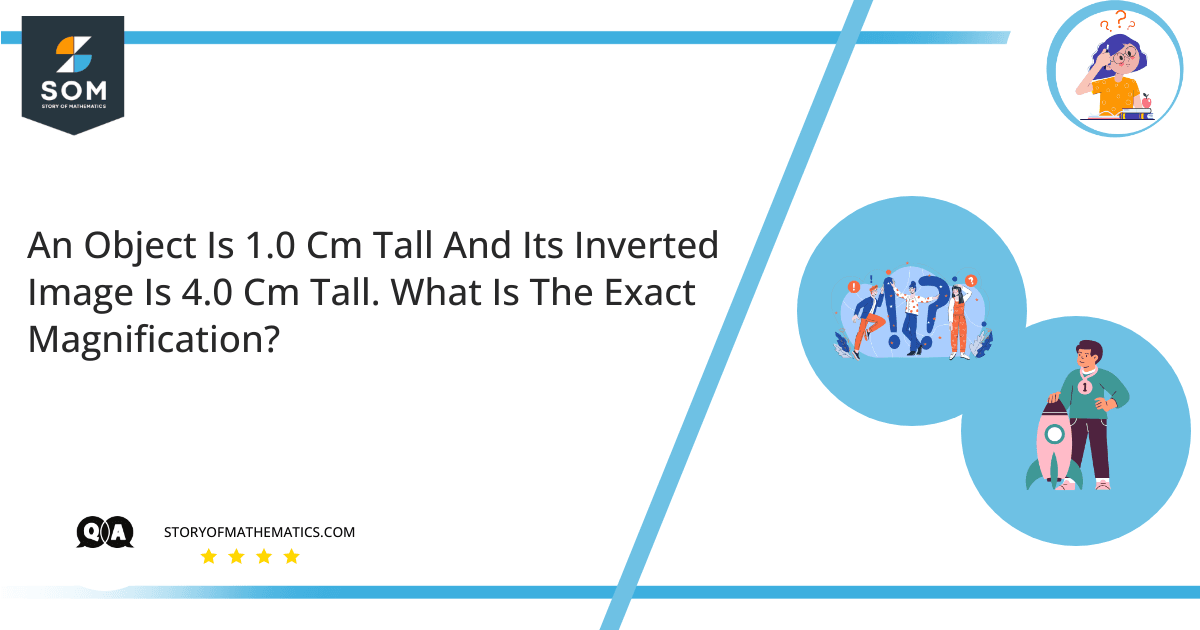# An object is 1.0 cm tall and its inverted image is 4.0 cm tall. What is the exact magnification?The main objective of this question is to find the lens magnification.This question uses the concept of lens magnification. Lens magnification is the ratio between the height of the image and the height of the object. It is mathematically represented as:$m \space = \space \frac{h_i}{h_o}$Where the lens magnification is m and h_i is the height of the image and h_o is the height of the object.

We are given:

Height of the object, $h_o = 1.0 cm$.

Height of the image, $h_o = \space 4.0 cm$.

We have to find the lens magnification.

We know that:

$m \space = \space \frac{h_i}{h_o}$

Where the lens magnification is m and h_i is the height of the image and h_o is the height of the object.

By putting the values, we get:

$m \space = \space \frac{-4}{1}$

We place a minus sign with image height as it shows that the image is inverted.

$m \space = \space -4 \space$

Thus, the lens magnification is $-4$.

The lens magnification is $-4$ when the height of the image is $4 cm$ and the height of the object is $1 cm$.

## Example

Find the lens magnification when the height of the object is $1 cm$ and the height of the image is $5 cm$, $8 cm$, and $10 cm$.

We are given:

Height of the object, $h_o \= 1.0 cm$.

Height of the image, $h_o = 5.0 cm$.

We have to find the lens magnification.

We know that:

$m \space = \space \frac{h_i}{h_o}$

Where the lens magnification is m and h_i is the height of the image and h_o is the height of the object.

By putting the values, we get:

$m \space = \space \frac{-5}{1}$

We place a minus sign with image height as it shows that the image is inverted.

$m \space = \space -5 \space$

Thus, the lens magnification is $-5$.

Now solving for the image height of $8 cm$.

We are given that:

Height of the object, $h_o = 1.0 cm$.

Height of the image, $h_o = 8.0 cm$.

We have to find the lens magnification.

We know that:

$m \space = \space \frac{h_i}{h_o}$

Where the lens magnification is m and h_i is the height of the image and h_o is the height of the object.

By putting the values, we get

$m \space = \space \frac{-8}{1}$

We place a minus sign with image height as it shows that the image is inverted.

$m \space = \space -8 \space$

Thus the lens magnification is $-8$.

Now solving for the image height of $10 cm$.

We are given that:

Height of the object, $h_o = 1.0 cm$.

Height of the image, $h_o = 10.0 cm$.

$m \space = \space \frac{-10}{1}$

We place a minus sign with image height as it shows that the image is inverted.

$m \space = \space -10 \space$

Thus, the lens magnification is $-10$.